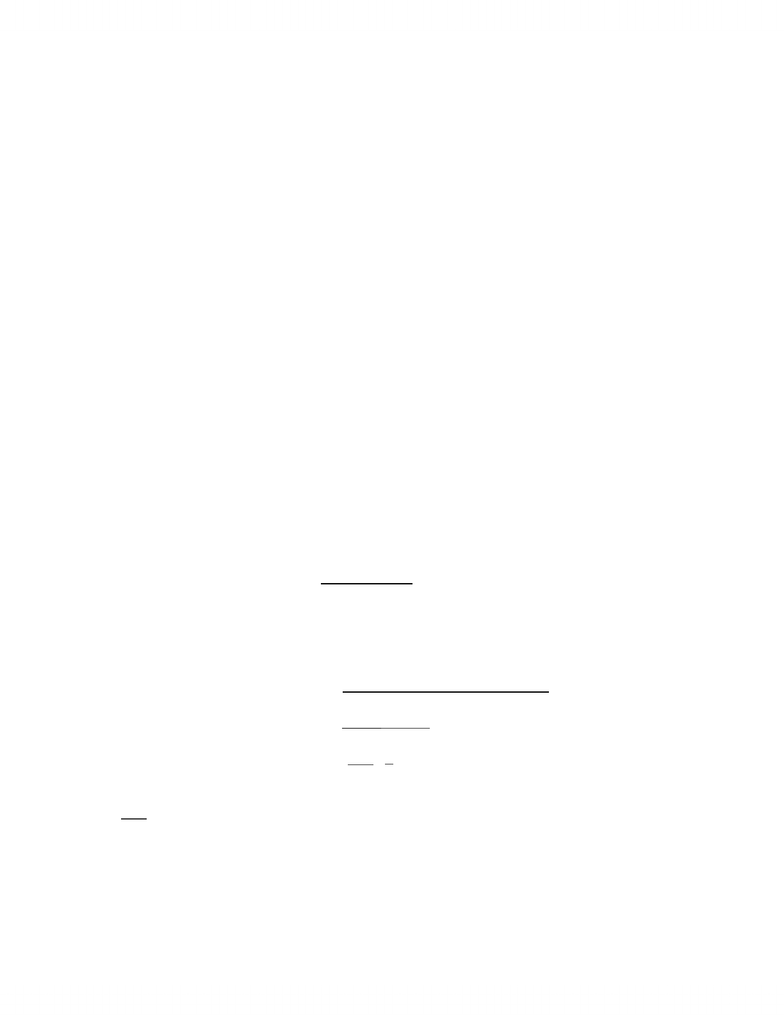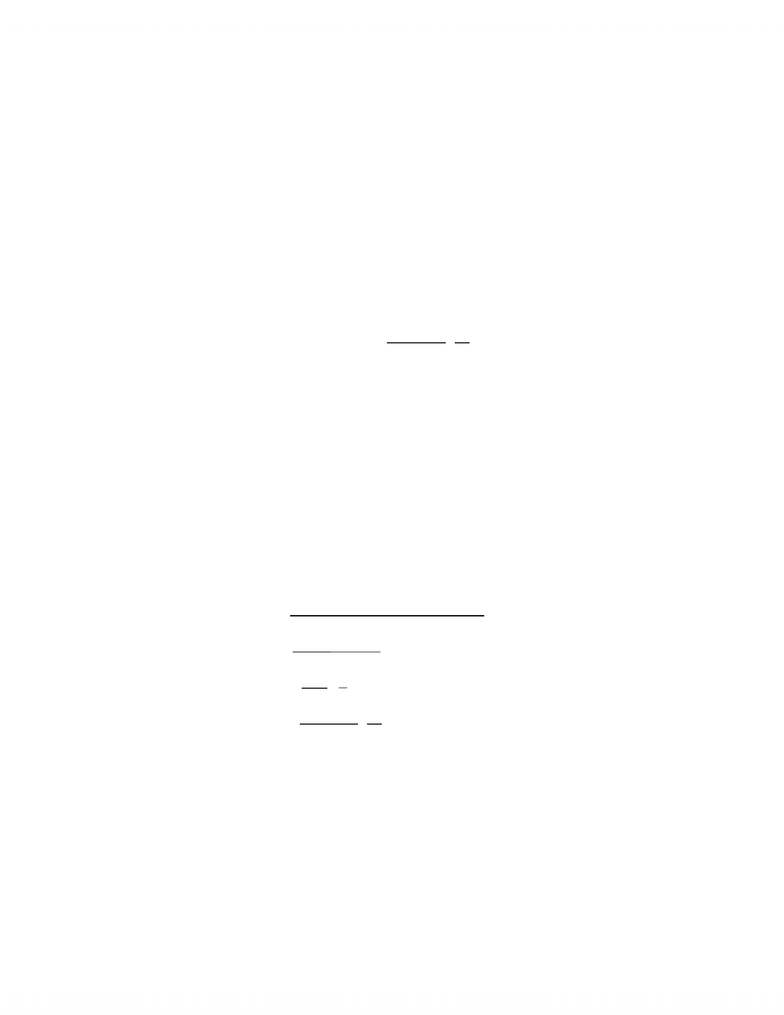# ECON 2310 Study Guide - Final Guide: Profit Maximization, Isoquant, Giffen Good

324 views40 pages
School
University of Guelph
Department
Economics
Course
ECON 2310Econ Notes for Final- 2310
Chapter 2
Demand curve shows how much buyers of the product want to buy at each
possible price, holding fixd all other factors that affect demand
Factors that affect the demand for a product include population growth
consumer tastes, incomes, the price of other products and government taxes
or regulations. a change in any of these factors will cause the entire demand
curve to shift
A change in price results in a shift along the demand curve.
Substitutes two products that if all else is equal, an increase in the price of one of
the products cases buyers to demand more of the other product (eg potatoes and
corn)
Complements two products that if all else is equal, an increase in the price of one
of the products causes consumers to demand less of the other product. (eg butter
and corn)
Demand function describes the amount of the product that is demanded for each
possible comination of its price and other factors
Q= D(Price, other factors)
Q = 14 2P
Inverse demand function how much the firm must charge to sell any given
quantity of its product
P= P(Quantity Demanded)
P= 7 Q/2
Supply Curve shows how much sellers of a product want to sell at each possible
price holding fixed all other factors that affect supply
Factors that affect supply of a product include technology, the price of inputs
the price tof other possible outputs and government taxes or regulations. A
change in one of these factors will result in the supply cure shifting
A change in price will cause movement along the supply curve
Supply function describes the amount of the prodct that is supplied for each
possible combination of it’s price and other factors
Unlock document

This preview shows pages 1-3 of the document.
Unlock all 40 pages and 3 million more documents.Q = S(Price, Other factors)
Q= 5p 6
Equilibrium price - the price at which the amounts supplied and demanded are
equal graphically it is the point where the demand curve and supply curve meet.
When there is an increase in demand (demand curve shift to the right) Price
will increase and quantity will increase
When there is a decrease in demand (demand curve shift to the left) Price
will decrease and quantity will decrease
When there is an increase in supply (supply cure shift to the right) price will
decrease and quantity will increase
When there is a decrease in supply (supply curve shift to the left) price will
increase and quantity will decrease.
When demand and supply increase, price is unknown and quantity rises
When demand and supply decrease, price is unknown and quantity falls
When demand increases and supply decreases, price rises and quantity is
unknown
When demand dicreases and supply increases price falls and quantity is
unkown
Elasticity the measure of the responsiveness of one ariable to changes in another
variable
E= % change in Y
% change in X
Price elasticity of demand change in the amount demanded for each percent
increase in the price
E(D)= % change in amount demanded
%change in price
=100x(Q/Q)
100x(P/P)
= (Q)(P)
(P)(Q)
(Q) = change in the amount demanded for each dollar that the price increases, it
(P) can also be refered to as B
E(D)= -B(P/Q)
Unlock document

This preview shows pages 1-3 of the document.
Unlock all 40 pages and 3 million more documents.Elastic when the elasticity of demand is less than negative one. The percentage
change in amount demanded is larger than the percentage change in price.
Inelastic when the elasticity of demand is greater than -1 but smaller than 0.
Percentage change in the amount demanded is smaller than thepercentage change
in price
Perfectly elastic when the demand curve is horizantal so the demand equals
negative infinity
Perfectly inelastic when the demand curve is vertical so that the elasticity of
demand is zero.
Elasticity for non linear demand curve = ( 1 ) (P)
(P/Q)(Q)
Constant elasticity/ isoelastic demand curve has the same elasticity at every
price
A small increase in price causes total expenditure to increase if demand is
inelastic
A small increase in price causes total expenditure to decrease if demand is
elastic
Total expenditure is largest at a price for which the elasticity equals -1
Elasticity of supply the responsiveness of supply to a products price
E(S)=% change in amount supplied
% change in price
=100x(Q/Q)
100x(P/P)
= (Q)(P)
(P)(Q)
E(S) =( 1 ) (P)
(P/Q)(Q)
perfectly inelastic supply - when the supply curve is vertical so that the price
elasticity of supply is zero
perfectly elastic supply when the supply curve is horizantal so that the price
elasticity of supply is infinite
elastic supply - at price when the elasticity of supply is greater than one
Unlock document

This preview shows pages 1-3 of the document.
Unlock all 40 pages and 3 million more documents.

## Document Summary

Demand curve shows how much buyers of the product want to buy at each possible price, holding fixd all other factors that affect demand. Factors that affect the demand for a product include population growth consumer tastes, incomes, the price of other products and government taxes or regulations. A change in any of these factors will cause the entire demand curve to shift. A change in price results in a shift along the demand curve. Substitutes two products that if all else is equal, an increase in the price of one of the products cases buyers to demand more of the other product (eg potatoes and corn) Complements two products that if all else is equal, an increase in the price of one of the products causes consumers to demand less of the other product. (eg butter and corn) Demand function describes the amount of the product that is demanded for each possible comination of its price and other factors.